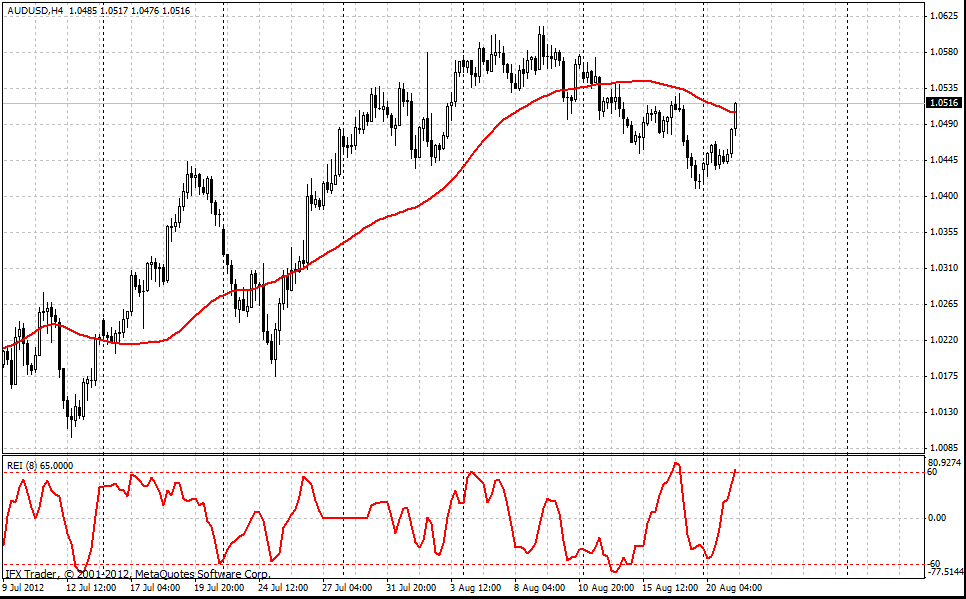# रेंज विस्तार सूचकांक

Technical indicator Range Expansion Index was developed by Thomas DeMark and presented in his book The New Science of Technical Analysis (Thomas R. DeMark, The New Science of Technical Analysis, John Wiley&Sons, Inc., 1994). This is a technical indicator, namely, an oscillator, which can be applied on currency, stock and commodity markets.

#### Formula

Two sums are calculated for each day. One is the conditional sum of the "strong" price changes:

S1 = Sum(k, j=1) n(j)m(j)s(j)

where "k" is the period of calculation (usually, 8), is a first condition:

if ((High[j - 2] < Close[j - 7]) && (High[j - 2] < Close[j - 8]) && (High[j] < High[j - 5]) && (High[j] < High[j - 6])) n(j) = 0, else n (j) = 1

m(j) is a second condition:

if ((Low[j - 2] > Close[j - 7]) && (Low[j - 2] > Close[j - 8]) && (Low[j] > Low[j - 5]) && (Low[j] > Low[j - 6])) m(j) = 0, else m(j) = 1

and s(j) is the price change parameter:

s(j) = High[j]-High[j-2]+Low[j] - Low[j-2]

The second sum is calculated as following:

S(2) = Sum(k, j=1) |High[j]-High[j-2]|+|Low[j] - Low[j-2]|

For each trading day the value of the indicator is calculated:

REI = S1/S2*100

The Range Expansion index can be used in short-term and long-term timeframes as an extra oscillator indicating likely extreme price movements.

This indicator fluctuates within the range from +100 to -100 percent. Its values running over +60 mean overbought market conditions and a possible price reversal in the short time. The indicator values above +60 suggest that purchases dominate. When REI drops below the -60 level, it indicates that the price considerably fell in a given timeframe and a rebound is possible.

Interestingly, the indicator remains in the neutral zone on the flat market (when the price is moving sideways): in the range from -60 to +60. This feature was added to the indicator by its developer Thomas DeMark. It effectively works on today's financial markets: if there is a sideways movement, then REI will not generate extremes in the overbought or oversold zones, so it will not produce false reversal signals when they might be created by the market.

If the indicator is used below H4 timeframes, the standard settings are recommended to be increased from 8 to 12 in order to cut down false signals and noise.

Trading based on the indicator's signals (extremes in the overbought or oversold zones) should include the filter selecting the signals being formed. One of the simplest and relevant methods of filtering is the use of 50 period Simple MA. In this connection, the following trading scheme should be applied:

- A short-term position is opened if REI generates the extreme above the +60 level with the price being below SMA (50).
- A long-term position is opened if REI generates the extreme below the -60 level with the price being above SMA (50).

Such a filter reduces the total number of deals almost by one third, excluding trades against mid-term trend however. This has a positive effect on the result of trades.#### InstaForex REI parameters

REI_period = 8

अभी बात नहीं कर सकते?
अपना प्रश्न पूछें बातचीत.